# Page Replacement Algorithm Notes | Study Operating System - Computer Science Engineering (CSE)

## Computer Science Engineering (CSE): Page Replacement Algorithm Notes | Study Operating System - Computer Science Engineering (CSE)

The document Page Replacement Algorithm Notes | Study Operating System - Computer Science Engineering (CSE) is a part of the Computer Science Engineering (CSE) Course Operating System.
All you need of Computer Science Engineering (CSE) at this link: Computer Science Engineering (CSE)

Optimal Page Replacement Algorithm

In operating systems, whenever a new page is referred and not present in memory, page fault occurs and Operating System replaces one of the existing pages with newly needed page. Different page replacement algorithms suggest different ways to decide which page to replace. The target for all algorithms is to reduce number of page faults.
In this algorithm, OS replaces the page that will not be used for the longest period of time in future.

Examples:
Input : Number of frames, fn = 3
Reference String, pg[] = {7, 0, 1, 2,
0, 3, 0, 4, 2, 3, 0, 3, 2, 1,
2, 0, 1, 7, 0, 1};
Output : No. of hits = 11
No. of misses = 9
Input : Number of frames, fn = 4
Reference String, pg[] = {7, 0, 1, 2,
0, 3, 0, 4, 2, 3, 0, 3, 2};
Output : No. of hits = 7
No. of misses = 6

The idea is simple, for every reference we do following:

1. If referred page is already present, increment hit count.
2. If not present, find if a page that is never referenced in future. If such a page exists, replace this page with new page. If no such page exists, find a page that is referenced farthest in future. Replace this page with new page.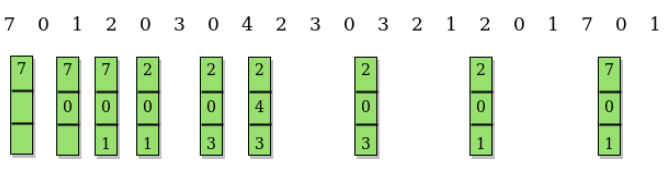Second Chance (or Clock) Page Replacement Policy

Apart from LRU, OPT and FIFO page replacement policies, we also have the second chance/clock page replacement policy. In the Second Chance page replacement policy, the candidate pages for removal are considered in a round robin matter, and a page that has been accessed between consecutive considerations will not be replaced. The page replaced is the one that, when considered in a round robin matter, has not been accessed since its last consideration.
It can be implemented by adding a “second chance” bit to each memory frame-every time the frame is considered (due to a reference made to the page inside it), this bit is set to 1, which gives the page a second chance, as when we consider the candidate page for replacement, we replace the first one with this bit set to 0 (while zeroing out bits of the other pages we see in the process). Thus, a page with the “second chance” bit set to 1 is never replaced during the first consideration and will only be replaced if all the other pages deserve a second chance too!

Example:
Let’s say the reference string is 0 4 1 4 2 4 3 4 2 4 0 4 1 4 2 4 3 4 and we have 3 frames. Let’s see how the algorithm proceeds by tracking the second chance bit and the pointer.

• Initially, all frames are empty so after first 3 passes they will be filled with {0, 4, 1} and the second chance array will be {0, 0, 0} as none has been referenced yet. Also, the pointer will cycle back to 0.
• Pass-4: Frame={0, 4, 1}, second_chance = {0, 1, 0} [4 will get a second chance], pointer = 0 (No page needed to be updated so the candidate is still page in frame 0), pf = 3 (No increase in page fault number).
• Pass-5: Frame={2, 4, 1}, second_chance= {0, 1, 0} [0 replaced; it’s second chance bit was 0, so it didn’t get a second chance], pointer=1 (updated), pf=4
• Pass-6: Frame={2, 4, 1}, second_chance={0, 1, 0}, pointer=1, pf=4 (No change)
• Pass-7: Frame={2, 4, 3}, second_chance= {0, 0, 0} [4 survived but it’s second chance bit became 0], pointer=0 (as element at index 2 was finally replaced), pf=5
• Pass-8: Frame={2, 4, 3}, second_chance= {0, 1, 0} [4 referenced again], pointer=0, pf=5
• Pass-9: Frame={2, 4, 3}, second_chance= {1, 1, 0} [2 referenced again], pointer=0, pf=5
• Pass-10: Frame={2, 4, 3}, second_chance= {1, 1, 0}, pointer=0, pf=5 (no change)
• Pass-11: Frame={2, 4, 0}, second_chance= {0, 0, 0}, pointer=0, pf=6 (2 and 4 got second chances)
• Pass-12: Frame={2, 4, 0}, second_chance= {0, 1, 0}, pointer=0, pf=6 (4 will again get a second chance)
• Pass-13: Frame={1, 4, 0}, second_chance= {0, 1, 0}, pointer=1, pf=7 (pointer updated, pf updated)
• Page-14: Frame={1, 4, 0}, second_chance= {0, 1, 0}, pointer=1, pf=7 (No change)
• Page-15: Frame={1, 4, 2}, second_chance= {0, 0, 0}, pointer=0, pf=8 (4 survived again due to 2nd chance!)
• Page-16: Frame={1, 4, 2}, second_chance= {0, 1, 0}, pointer=0, pf=8 (2nd chance updated)
• Page-17: Frame={3, 4, 2}, second_chance= {0, 1, 0}, pointer=1, pf=9 (pointer, pf updated)
• Page-18: Frame={3, 4, 2}, second_chance= {0, 1, 0}, pointer=1, pf=9 (No change)

In this example, second chance algorithm does as well as the LRU method, which is much more expensive to implement in hardware.

More Examples:
Input: 2 5 10 1 2 2 6 9 1 2 10 2 6 1 2 1 6 9 5 1
3
Output: 13

Input: 2 5 10 1 2 2 6 9 1 2 10 2 6 1 2 1 6 9 5 1
4
Output: 11

Algorithm:
Create an array frames to track the pages currently in memory and another Boolean array second_chance to track whether that page has been accessed since it’s last replacement (that is if it deserves a second chance or not) and a variable pointer to track the target for replacement.

1. Start traversing the array arr. If the page already exists, simply set its corresponding element in second_chance to true and return.
2. If the page doesn’t exist, check whether the space pointed to by pointer is empty (indicating cache isn’t full yet) – if so, we will put the element there and return, else we’ll traverse the array arr one by one (cyclically using the value of pointer), marking all corresponding second_chance elements as false, till we find a one that’s already false. That is the most suitable page for replacement, so we do so and return.
3. Finally, we report the page fault count.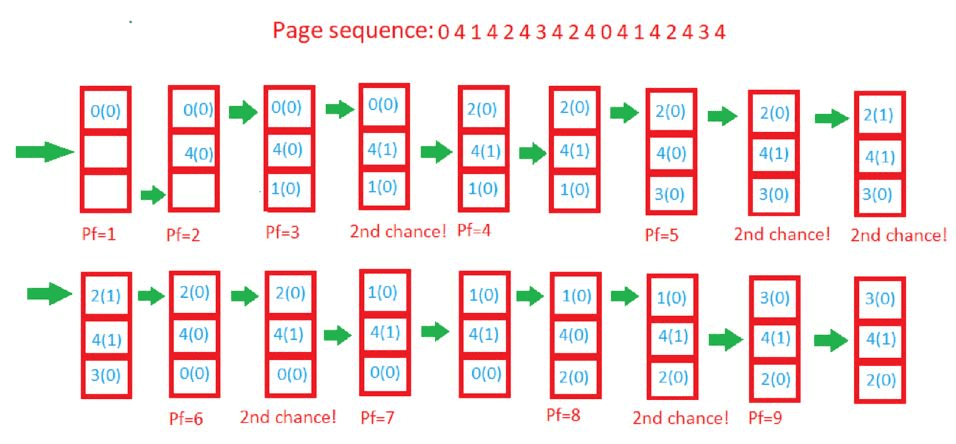Belady’s Anomaly in Page Replacement Algorithms

In Operating System, process data is loaded in fixed sized chunks and each chunk is referred to as a page. The processor loads these pages in the fixed sized chunks of memory called frames. Typically the size of each page is always equal to the frame size.
A page fault occurs when a page is not found in the memory, and needs to be loaded from the disk. If a page fault occurs and all memory frames have been already allocated, then replacement of a page in memory is required on the request of a new page. This is referred to as demand-paging. The choice of which page to replace is specified by a page replacement algorithms. The commonly used page replacement algorithms are FIFO, LRU, optimal page replacement algorithms etc.
Generally, on increasing the number of frames to a process’ virtual memory, its execution becomes faster as less number of page faults occur. Sometimes the reverse happens, i.e. more number of page faults occur when more frames are allocated to a process. This most unexpected result is termed as Belady’s Anomaly.
Bélády’s anomaly is the name given to the phenomenon where increasing the number of page frames results in an increase in the number of page faults for a given memory access pattern.

This phenomenon is commonly experienced in following page replacement algorithms:

1. First in first out (FIFO)
2. Second chance algorithm
3. Random page replacement algorithm

The other two commonly used page replacement algorithms are Optimal and LRU, but Belady’s Anamoly can never occur in these algorithms for any reference string as they belong to a class of stack based page replacement algorithms.
A stack based algorithm is one for which it can be shown that the set of pages in memory for N frames is always a subset of the set of pages that would be in memory with N + 1 frames. For LRU replacement, the set of pages in memory would be the n most recently referenced pages. If the number of frames increases then these n pages will still be the most recently referenced and so, will still be in the memory. While in FIFO, if a page named b came into physical memory before a page – a then priority of replacement of b is greater than that of a, but this is not independent of the number of page frames and hence, FIFO does not follow a stack page replacement policy and therefore suffers Belady’s Anomaly.
Example: Consider the following diagram to understand the behaviour of a stack-based page replacement algorithm.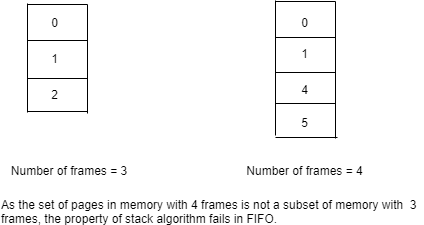The diagram illustrates that given the set of pages i.e. {0, 1, 2} in 3 frames of memory is not a subset of the pages in memory – {0, 1, 4, 5} with 4 frames and it is a violation in the property of stack based algorithms. This situation can be frequently seen in FIFO algorithm.

Assuming a system that has no pages loaded in the memory and uses the FIFO Page replacement algorithm. Consider the following reference string:
1, 2, 3, 4, 1, 2, 5, 1, 2, 3, 4, 5
Case-1: If the system has 3 frames, the given reference string on using FIFO page replacement algorithm yields a total of 9 page faults. The diagram below illustrates the pattern of the page faults occurring in the example.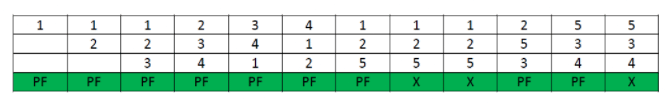Case-2: If the system has 4 frames, the given reference string on using FIFO page replacement algorithm yields a total of 10 page faults. The diagram below illustrates the pattern of the page faults occurring in the example.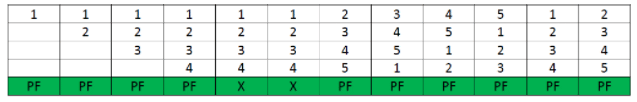It can be seen from the above example that on increasing the number of frames while using the FIFO page replacement algorithm, the number of page faults increased from 9 to 10.

Note: It is not necessary that every string reference pattern cause Belady anomaly in FIFO but there are certain kind of string references that worsen the FIFO performance on increasing the number of frames.

Why Stack based algorithms do not suffer Anomaly
All the stack based algorithms never suffer Belady Anomaly because these type of algorithms assigns a priority to a page (for replacement) that is independent of the number of page frames. Examples of such policies are Optimal, LRU and LFU. Additionally these algorithms also have a good property for simulation, i.e. the miss (or hit) ratio can be computed for any number of page frames with a single pass through the reference string.
In LRU algorithm every time a page is referenced it is moved at the top of the stack, so, the top n pages of the stack are the n most recently used pages. Even if the number of frames are incremented to n+1, top of the stack will have n+1 most recently used pages.
Similar example can be used to calculate the number of page faults in LRU algorithm.
Assuming a system that has no pages loaded in the memory and uses the LRU Page replacement algorithm. Consider the following reference string:
1, 2, 3, 4, 1, 2, 5, 1, 2, 3, 4, 5
Case-1: If the system has 3 frames, the given reference string on using LRU page replacement algorithm yields a total of 10 page faults. The diagram below illustrates the pattern of the page faults occurring in the example.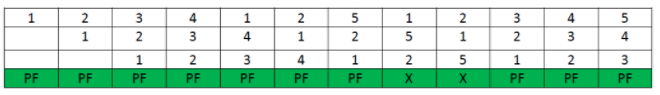Case-2: If the system has 4 frames, the given reference string on using LRU page replacement algorithm, then total 8 page faults occur. The diagram shows the pattern of the page faults in the example.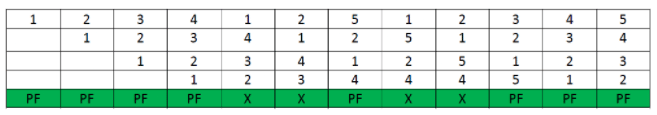Conclusion:
Various factors substantially affect the number of page faults, such as reference string length and the number of free page frames available. Anomalies also occurs due to the small cache size as well as the reckless rate of change of the contents of cache. Also, the situation of fixed number of page faults even after increasing the number of frames can also be seen as an anomaly. Often algorithms like Random page replacement algorithm are also susceptible to Belady’s Anomaly, because it may behave like first in first out (FIFO) page replacement algorithm. But Stack based algorithms are generally immune to all such situations as they are guaranteed to give better page hits when the frames are incremented.

The document Page Replacement Algorithm Notes | Study Operating System - Computer Science Engineering (CSE) is a part of the Computer Science Engineering (CSE) Course Operating System.
All you need of Computer Science Engineering (CSE) at this link: Computer Science Engineering (CSE)Use Code STAYHOME200 and get INR 200 additional OFF

## Operating System

69 docs|23 tests

Track your progress, build streaks, highlight & save important lessons and more!

,

,

,

,

,

,

,

,

,

,

,

,

,

,

,

,

,

,

,

,

,

;